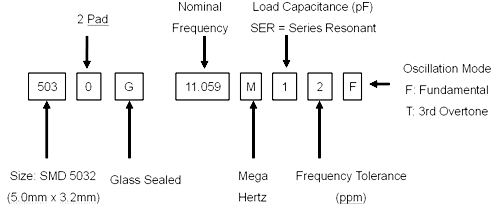Technical Information

• Quartz cut angle

• Quartz blank dimension

• Saw Wafer Dimension

• Synthetic Quartz Crystal Bars

• Quartz Crystals

Quartz, composed of cilicon and Oxygen (silicon Dioxide), exhibit piezoelectricity Property. This device generate an electrical potenial when pressure is applied on The surface of the crystal. Inversely, when an electrical potential is applied to the syurface of a crystal, mechanical deformation or vibration is generated.

• Type of Quartz Crystals

Quartz crystal (crystal units) are produced from crystal elements cut at a direction Predetermined in accordance with the purpose for which they will be used. Crystal units should have the follow specifications:
1), Equivalent circuit and paramenters
2), Characteristics of frequency vs load capacitance.
3), Characteristics of frequency vs temperature.

• Equivalent Circuit Parameters

• Equivalent series resistance (R1)

The resistance of the crystal unit alone at the resonant frequency.

• Motional inductance (L1)

The inductance in the motional (series) arm of the equivalent circuit.

• Motional capacitance (C1)

The capacitance of the motional (series) arm of the equivalent circuit.

• Shunt capacitance (C0)

The static capacitance between the electrodes, together with stray capacitance of the mounting system

• Capacitance ration (R)

The ratio of the shunt capacitance to the motional capacitance
R = C0 / C1

• Series resonant frequency of quartz blank (FS)

The lower of the two frequencies of the crystal unit alone, under specified conditions, at which the electrical impedance of the crystal unit is resistive<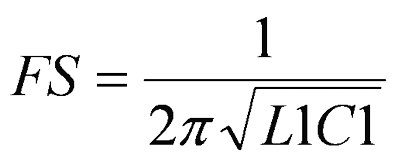• Load resonant frequency (FL)

One of the two frequencies of a crystal unit in association with a series or with a parallel load capacitance, under specified conditions, at which the electrical impedance of the combination is resistive. The frequency is the lowest of the two frequencies when the load capacitance is in series.• Quality factor (Q)

Ln a resonant circuit composed of an L, C and R, a quantity that represents the sharpness of a resonant curve that shows the relationship between the circuit current and power frequency.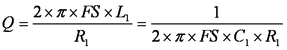• Trim sensitive of load measure (TS)

The increment fractional frequency change for an increment change in the load capacitance.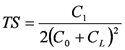• Spurious

Frequency responses other than the main or desired response, which the crystal elements have/• Pullability

A specification for the change in frequency (express in ppm) for a “parallel load resonant” crystal as a function of change in load capacitance (expressed in pF)

1)Load resonant to series resonant (PPM)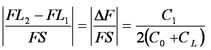2)Load resonant 1 to load resonant 2 (PPM)• Drive Level (DL)

The level of power or current in the crystal unit when in the operating statel: current to pass in the quartz crystal
Re: effective resistance of quartz crystal

• Allowance for oscillation

Unless adequate negative resistance is allocation in the oscillation circuit, startup time of oscillation may be increased or no oscillation may occur. In order to avoid this, enough negative resistance in the circuit design should be provided.

• Negative resistance (-R)

The negative resistance, also refer to as the degree of oscillation allowance that can be used for judging the quality of the circuit side oscillation motivity. The use of a circuit with an insufficient negative resistance may lead to such an unexpected trouble as the quartz crystal unit failing to initiate oscillation even when power has been switched on.

1) connect the resistance (R) to the circuit in series with the quartz crystal
2) adjust R so that oscillation can start (or stop)
3) measure R when oscillation just start (or stop) in above 2
4) get the negative resistance
-R = R + R1
5) Recommended l-R1
1-R1>Re*(5 to 10)

• Insulation resistance

Resistance between leads or between lead and the case

• Aging

The change in operating frequency over a specified time period and is typically expressed as a maximum value in parts per million per year (ppm/year). This frequency change as a function of time is due to many factors: seal method and integrity, manufacturing processes, material type, operation temperature and frequency of operation.

• Part Number System

SMD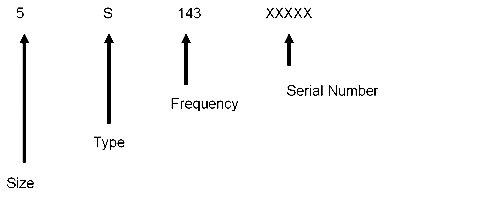Size Code Type Code
8045 8 Seam S
7050 7 Glass G
6035 6 OSC C
5032 5 TGXO T
4025 4
3225 3
2520 2
2016 9
1612 1

DTB

Type Code
49U U
49S S
49SDD/U4C M
UM series N
50U Series V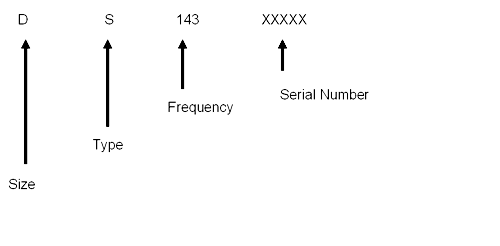Example